# 2. Compute the fixed overhead spendllg Exercise 10-59 Overhead Application, Fixed and Variable Ov...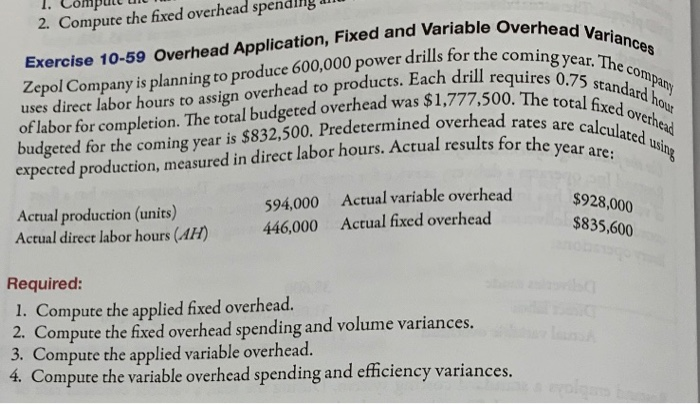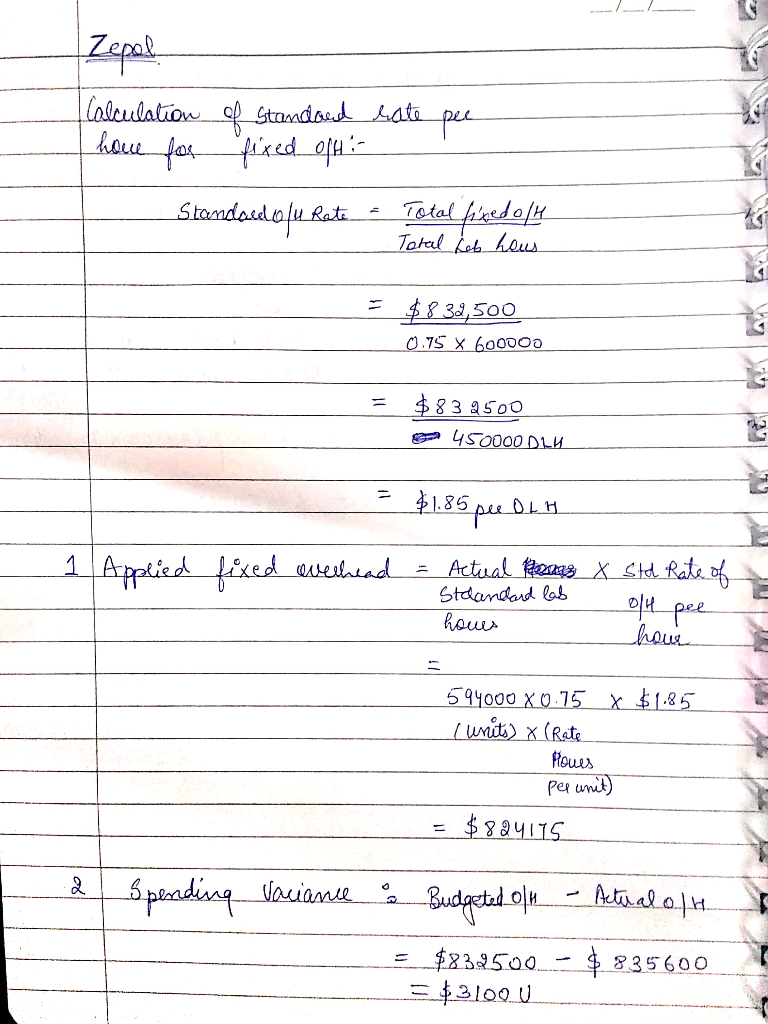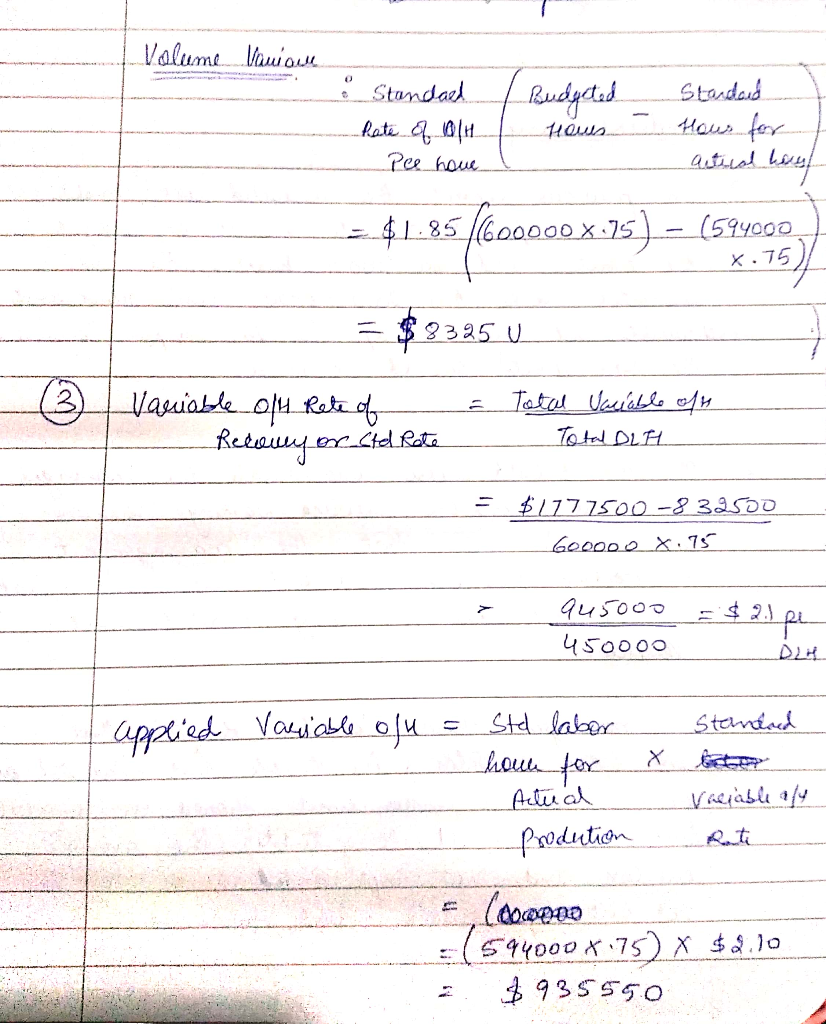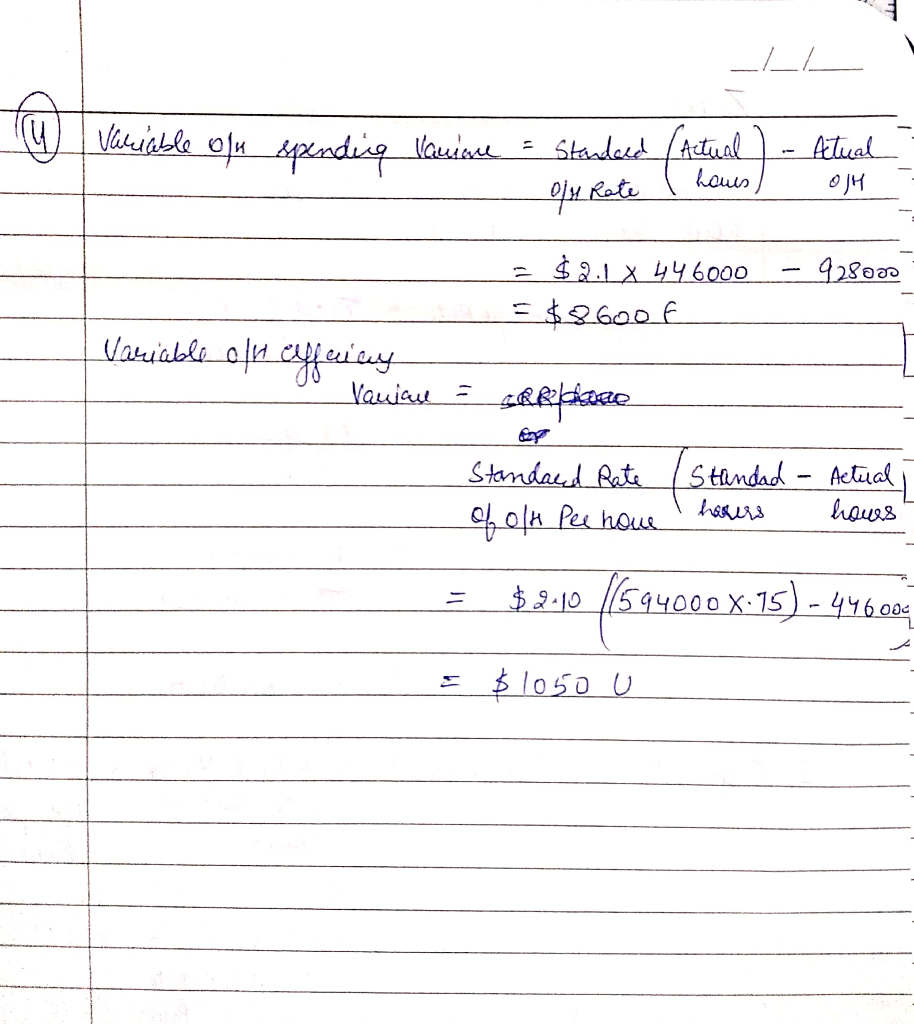Similar Homework Help Questions
• ### Overhead Application, Fixed and Variable Overhead Variances Zepol Company is planning to produce 600,000 power drills...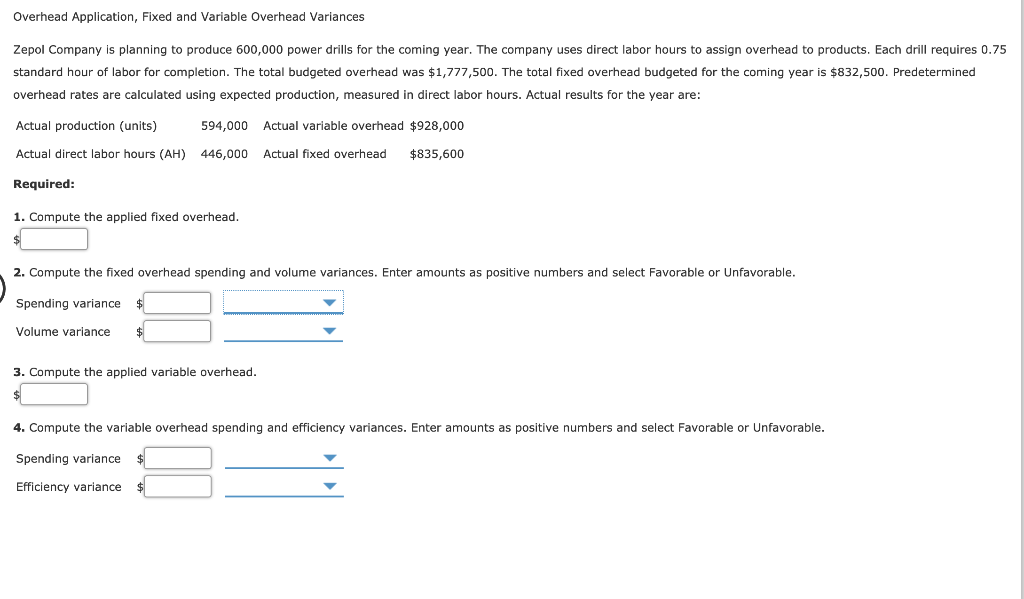Overhead Application, Fixed and Variable Overhead Variances Zepol Company is planning to produce 600,000 power drills for the coming year. The company uses direct labor hours to assign overhead to products. Each drill requires 0.75 standard hour of labor for completion. The total budgeted overhead was \$1,777,500. The total fixed overhead budgeted for the coming year is \$832,500. Predetermined overhead rates are calculated using expected production, measured in direct labor hours. Actual results for the year are: Actual production (units)...

• ### Overhead Application, Overhead Variances, Journal Entries Plimpton Company produces countertop ovens. Plimpton uses a standard costing...

Overhead Application, Overhead Variances, Journal Entries Plimpton Company produces countertop ovens. Plimpton uses a standard costing system. The standard costing system relies on direct labor hours to assign overhead costs to production. The direct labor standard indicates that two direct labor hours should be used for every oven produced. The normal production volume is 100,000 units. The budgeted overhead for the coming year is as follows: Fixed overhead \$770,000 Variable overhead 446,000* *At normal volume. Plimpton applies overhead on the...

• ### Overhead Variances, Four-Variance Analysis Oerstman, Inc., uses a standard costing system and develops its overhead rates...

Overhead Variances, Four-Variance Analysis Oerstman, Inc., uses a standard costing system and develops its overhead rates from the current annual budget. The budget is based on an expected annual output of 129,000 units requiring 516,000 direct labor hours. (Practical capacity is 536,000 hours.) Annual budgeted overhead costs total \$861,720, of which \$608,880 is fixed overhead. A total of 119,000 units using 514,000 direct labor hours were produced during the year. Actual variable overhead costs for the year were \$260,100, and...

• ### Overhead Variances, Four-Variance Analysis Oerstman, Inc., uses a standard costing system and develops its overhead rates...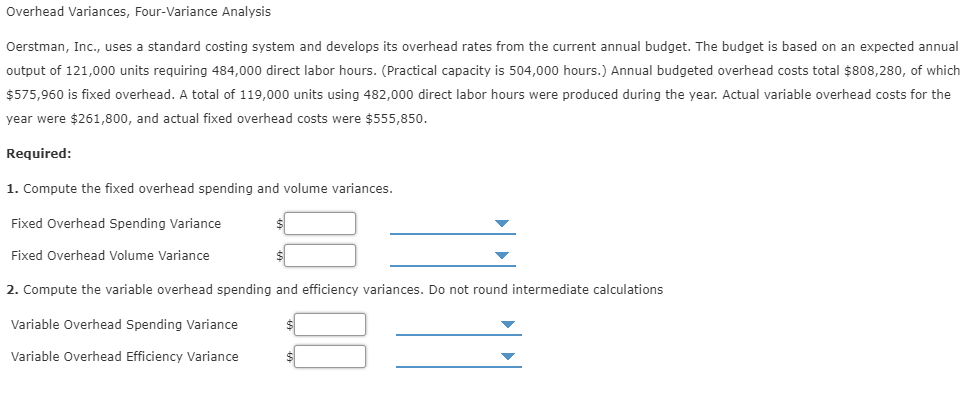Overhead Variances, Four-Variance Analysis Oerstman, Inc., uses a standard costing system and develops its overhead rates from the current annual budget. The budget is based on an expected annual output of 121,000 units requiring 484,000 direct labor hours. (Practical capacity is 504,000 hours.) Annual budgeted overhead costs total \$808,280, of which \$575,960 is fixed overhead. A total of 119,000 units using 482,000 direct labor hours were produced during the year. Actual variable overhead costs for the year were \$261,800, and...

• ### Overhead Variances, Four-Variance Analysis Oerstman, Inc., uses a standard costing system and develops its overhead rates...

Overhead Variances, Four-Variance Analysis Oerstman, Inc., uses a standard costing system and develops its overhead rates from the current annual budget. The budget is based on an expected annual output of 120,000 units requiring 480,000 direct labor hours. (Practical capacity is 500,000 hours.) Annual budgeted overhead costs total \$796,800, of which \$571,200 is fixed overhead. A total of 119,400 units using 478,000 direct labor hours were produced during the year. Actual variable overhead costs for the year were \$260,400, and...

• ### Overhead Variances, Four-Variance Analysis Oerstman, Inc., uses a standard costing system and develops its overhead rates...

Overhead Variances, Four-Variance Analysis Oerstman, Inc., uses a standard costing system and develops its overhead rates from the current annual budget. The budget is based on an expected annual output of 124,000 units requiring 496,000 direct labor hours. (Practical capacity is 516,000 hours.) Annual budgeted overhead costs total \$828,320, of which \$590,240 is fixed overhead. A total of 119,200 units using 494,000 direct labor hours were produced during the year. Actual variable overhead costs for the year were \$261,300, and...

• ### Overhead Variances, Four-Variance Analysis Oerstman, Inc., uses a standard costing system and develops its overhead rates...

Overhead Variances, Four-Variance Analysis Oerstman, Inc., uses a standard costing system and develops its overhead rates from the current annual budget. The budget is based on an expected annual output of 126,000 units requiring 504,000 direct labor hours. (Practical capacity is 524,000 hours.) Annual budgeted overhead costs total \$826,560, of which \$599,760 is fixed overhead. A total of 119,400 units using 502,000 direct labor hours were produced during the year. Actual variable overhead costs for the year were \$260,100, and...

• ### Overhead Variances, Four-Variance Analysis Oerstman, Inc., uses a standard costing system and develops its overhead rates...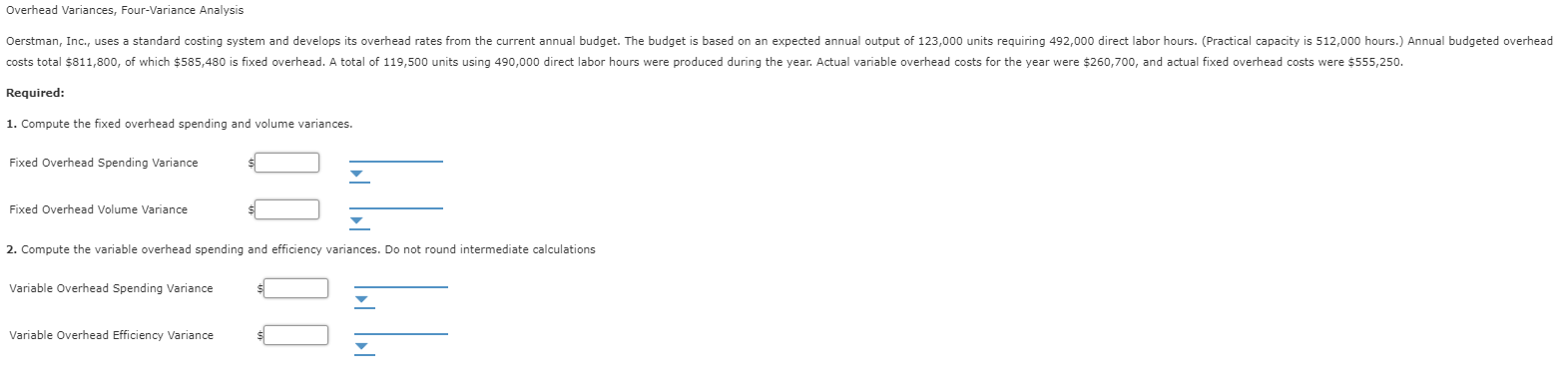Overhead Variances, Four-Variance Analysis Oerstman, Inc., uses a standard costing system and develops its overhead rates from the current annual budget. The budget is based on an expected annual output of 123,000 units requiring 492,000 direct labor hours. (Practical capacity is 512,000 hours.) Annual budgeted overhead costs total \$811,800, of which \$585,480 is fixed overhead. A total of 119,500 units using 490,000 direct labor hours were produced during the year. Actual variable overhead costs for the year were \$260,700, and...

• ### Overhead Variances, Four-Variance Analysis Oerstman, Inc., uses a standard costing system and develops its overhead rates...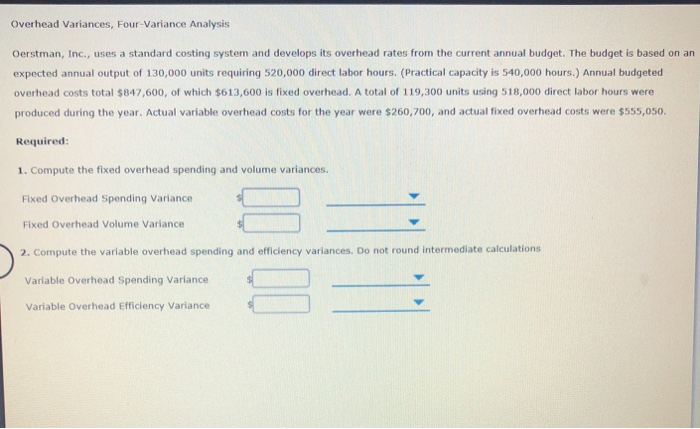Overhead Variances, Four-Variance Analysis Oerstman, Inc., uses a standard costing system and develops its overhead rates from the current annual budget. The budget is based on an expected annual output of 130,000 units requiring 520,000 direct labor hours. (Practical capacity is 540,000 hours.) Annual budgeted overhead costs total \$847,600, of which \$613,600 is fixed overhead. A total of 119,300 units using 518,000 direct labor hours were produced during the year. Actual variable overhead costs for the year were \$260,700, and...

• ### Overhead Variances, Four-Variance Analysis Oerstman, Inc., uses a standard costing system and develops its overhead rates...

Overhead Variances, Four-Variance Analysis Oerstman, Inc., uses a standard costing system and develops its overhead rates from the current annual budget. The budget is based on an expected annual output of 129,000 units requiring 516,000 direct labor hours. (Practical capacity is 536,000 hours.) Annual budgeted overhead costs total \$851,400, of which \$598,560 is fixed overhead. A total of 119,500 units using 514,000 direct labor hours were produced during the year. Actual variable overhead costs for the year were \$260,200, and...

Need Online Homework Help?